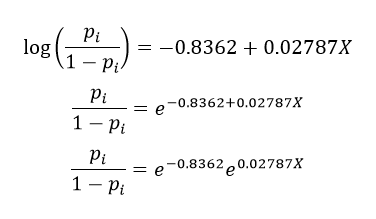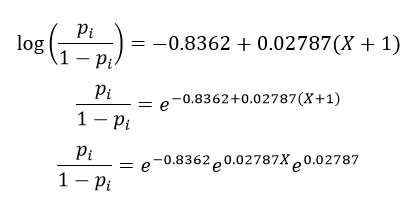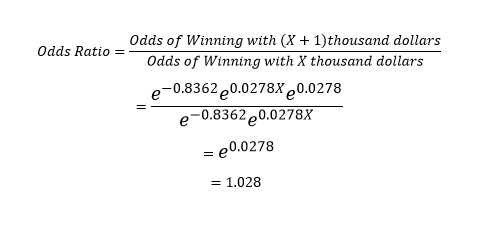# Odds Ratios

Details on the algebra involved with regards to interpreting logistic regression coefficients.

Suppose that a candidate raised X thousand dollars. Then the odds that they would win could be found as follows:Now suppose that a candidate raised (X+1) thousand dollars. Then the odds that they would win could be found as follows:Next, suppose that I wanted to find the odds ratio to compare a candidate who raised (X+1) thousand dollars with a candidate who raise X thousand dollars. One way to compare them, would be to look at the odds ratio as follows:Therefore, regardless of the value of X. The odds of candidate winning with X+1 thousand dollars raised would be 1.03 times higher than the odds of a candidate who raised X thousand dollars. That is, as X increases by 1 thousand dollars, the odds of winning the local election increases by a multiplicative factor of 1.03. This says that it is more likely to win with more money raised.